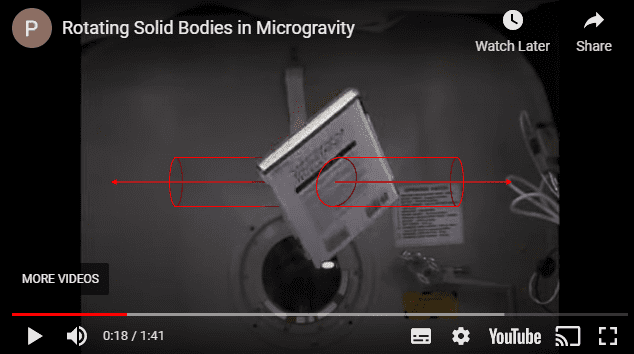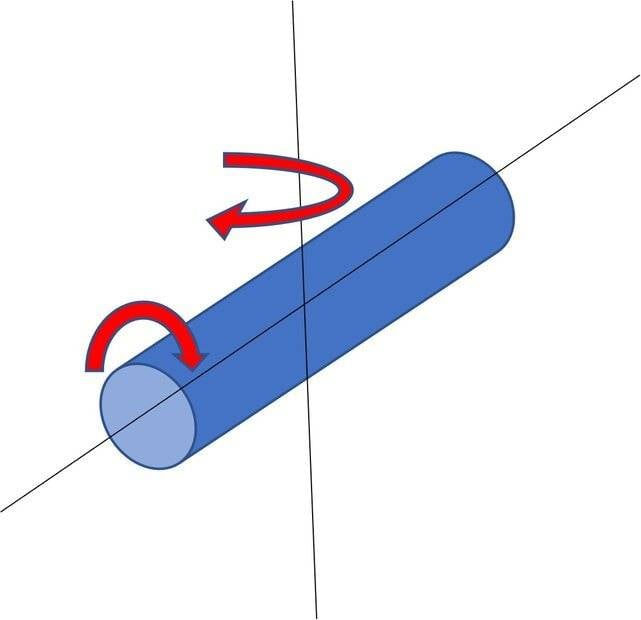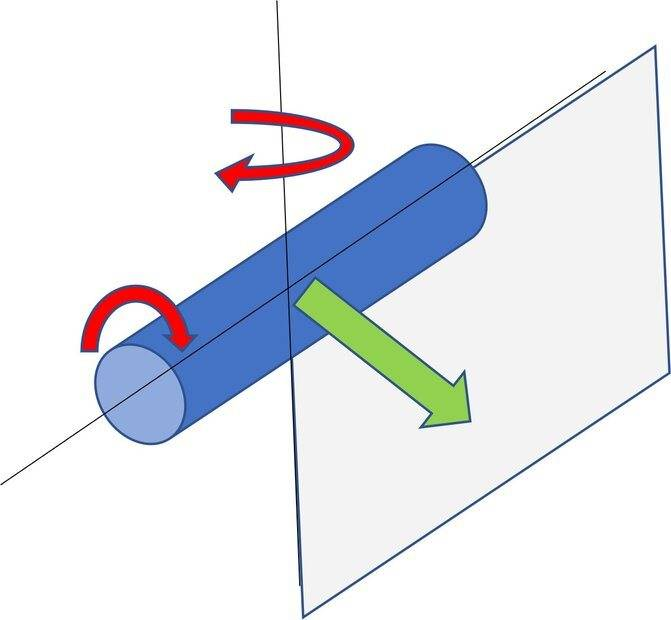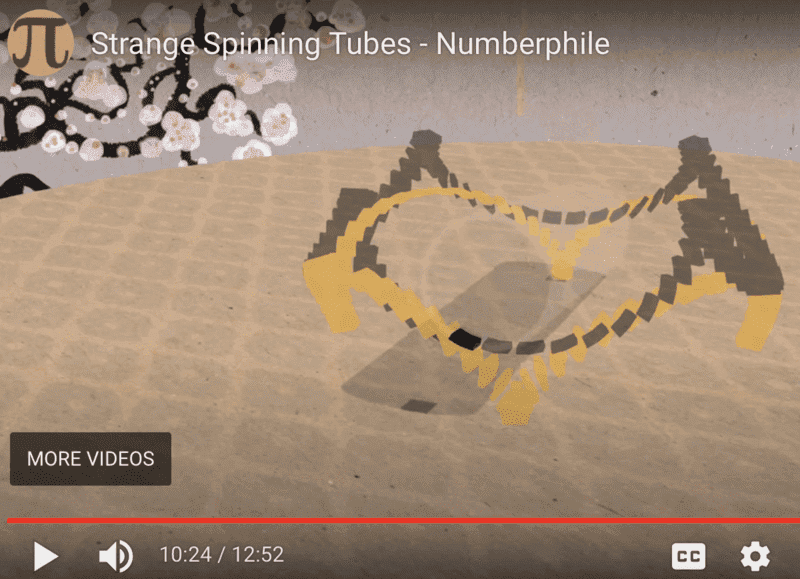# Artificial gravity rotating on two axes

Gold Member
TL;DR Summary
What would occupants experience inside a spaceship rotating on two axes?

An object can rotate on two axes simultaneously, yes? Is that stable in flat space?

If so, what would occupants experience as gravity? Would it change over time?

Gold Member
Are you imagining that if there were dwarfs in these bottles what would they experience ?

If they are floating in the hollow space in the bottle, they feel no gravity. The walls around are moving with no simple periodicity. If they have reference frame fixed with the walls, artificial gravity they feel would be complex one.

Last edited:
••Delta2 and DaveC426913
Gold Member
Yes, if their spaceship were rotating, eventually air drag will bring them to the floor. There,inertia would be experienced as ersatz gravity.

Homework Helper
2022 Award
For an object freely rotating there are two stable body axes of rotation (maximal an minimal moments of inertia). Other axes are unstable. So the rotating on two axes seems problematic.

Staff Emeritus
What does it even mean to be rotating about two axes? The angular momentum points in only one direction.

•Delta2, phinds, vanhees71 and 3 others
Gold Member
What does it even mean to be rotating about two axes? The angular momentum points in only one direction.
Gyroscopic precession is one way, I think. But can that happen in zero g without an applied force?

So, we take a stationary cigar-shaped spaceship, and set it spinning around its long axis.
Now we give one end a tap (or use attitude jets) to attempt to apply a rotation along a(ny) short axis.
What rotation does the ship get?

Is it always possible to sum these vectors to come up with a rotation about a single (albeit off-kilter) axis?

BTW, it is this thread/post that inspired this line of thought.

Last edited:
Gold Member

Yes. The book in this video, 0:17s - 0:28s, appears to be rotating about two axes simultaneously.

I'm not sure if that is an objective phenomenon, or if it only seems to be rotating about two axes because the shape of the book strongly implies three very un-arbitrary axes to our brains, and therefore it we track two axes.

So, does a single (albeit oblique) axis of rotation exist for this book?

(I think it does. I think it's here:)#### Attachments

Last edited:
bland
What does it even mean to be rotating about two axes? The angular momentum points in only one direction.

Yes, that would be like saying the Earth were rotating on multiple axes because of the wobble!

“The Bizarre Behavior of Rotating Bodies”.

Gold Member

These are spinning in two axis. I’m not sure if that is stable in space however. I’m thinking it “wants” to change axis of rotation but it can’t because of the table top?

•OmCheeto
Gold Member
“The Bizarre Behavior of Rotating Bodies”.
Yes. I've seen this, and have thought of it. I'm not sure it answer my question, but it might be useful to @Melbourne Guy

•Melbourne Guy
bland
This is what I thought the OP was originally asking. Imagine a very large enclosed gravity wheel turning in space at the correct speed, like the jogger on the space station on 2001 a Space Odyssey. Now imagine that the wheel was not turning but instead it was spinning on an axis that was a diameter of the wheel and the jogger was now standing still but the wheel was turning on the diameter axis at the correct speed such that it provided the correct artificial gravity such that the person could stand still on that spot.

Now, if I understand it correctly I think the OP was asking what would be the inertial forces on the person standing still if the wheel now also began rotating around it's original hub type rotation.

Homework Helper
Gold Member
2022 Award
Yes, that would be like saying the Earth were rotating on multiple axes because of the wobble!
If there isn't a single axis of rotation, then (by definition) the motion is not a rotation.

•Gold Member
A rigid object can (obviously) only have a single rotational vector at any given instance in time, but this rotation vector may not be parallel to any of the principal axes of the object in which case it is called non-pure rotation. Since "rotation around two axis at once" does not really make any sense taken literally, I guess one could take it to mean non-pure rotation, but then why not just call it that, i.e non-pure rotation. Alternatively one could say "rotation around a non-principal axis".

•russ_watters, jbriggs444 and PeroK
Gold Member
Since "rotation around two axis at once" does not really make any sense taken literally

I'm not understanding something, is this following scenario not considered rotation about two axis at once?••Delta2, OmCheeto and DaveC426913
Homework Helper
Gold Member
2022 Award
I'm not understanding something, is this following scenario not considered rotation about two axis at once?

View attachment 301451
If we combine a rotation of angular frequency ##w_x## about the x-axis and ##w_z## about the z-axis, then we have a rotation about the axis ##(w_x, 0, w_z)##, with magnitude ##\sqrt{w_x^2 + w_z^2}##.

In other words, the continuous rotations add like vectors.

•Delta2
Gold Member
is this following scenario not considered rotation about two axis at once
As mentioned, any rigid object can only have a single rotation vector at any given time, but it may very well be that such a vector is not parallel with the principal axis of the object. In your drawing that would be the black center line of the cylinder plus any two orthogonal axes in the plane through center of mass and orthogonal to the center line.

But perhaps you are asking why any rotation of a rigid body can be modeled as the rotation around a single axis? If so, the best quick answer I can come up with is Euler's rotation theorem which states that any sequence of spatial rotations can always be modeled as an equivalent rotation around a single axis. With a little hand-waving you can imagine that trying to rotate a free rigid object around two axis at once by applying two orthogonal torques will "combine" into a single resulting torque giving rotation around a single unique axis at any given time.

•DrClaude
Homework Helper
Gold Member
2022 Award
A few points to note.

1) If we ignore translation of the centre of mass of a rigid object, then any change to the object's position can only be a rotation.

2) As above, associated with any change in position there must be an axis and angle of rotation.

3) Although finite rotations do not commute, infinitesimal rotations do. Any continuous rotation, therefore, can be expressed as a vector ##\vec w = (w_x, w_y, w_z)##.

4) If, however, the components of ##\vec w## are changing continuously in different ways, then the resulting motion is not a continuous rotation - as the axis of rotation is changing with time. In which case, we can have continuous motion that is not a continuous rotation. (Even though at each instant we can find an instantaneous axis of rotation. And, the position of the rigid body at any time must be a rotation of its initial position).

•vanhees71 and Filip Larsen
Staff Emeritus
People seem confused by rotation. Is a body moving northeast going "in two directions at once"?

•Delta2, russ_watters, jbriggs444 and 1 other person
Gold Member
2022 Award
Maybe this basic primer about rigid-body dynamics helps:

https://itp.uni-frankfurt.de/~hees/pf-faq/spinning-top.pdf

The momentary rotation of the body is, of course, described by the momentary angular velocity, which is one vector. It doesn't make sense to say something is "rotating on two axes", it's just momentarily rotating around one axis given by the direction of the angular velocity.

•PeroK
Gold Member
People seem confused by rotation. Is a body moving northeast going "in two directions at once"?
No, it's just very hard to conceptualize - this:
If we combine a rotation of angular frequency ##w_x## about the x-axis and ##w_z## about the z-axis, then we have a rotation about the axis ##(w_x, 0, w_z)##, with magnitude ##\sqrt{w_x^2 + w_z^2}##.

In other words, the continuous rotations add like vectors.
I'm going to munge some things in my head for a second:

Let's say we have a body rotating once per second about the x-axis and once per ten seconds about the z-axis.
So there is a single axis a out which this object is rotating.

It is rotating about axis (1,0,10) with magnitude ##\sqrt{101}##.

Last edited:
Homework Helper
Gold Member
2022 Award
It is rotating about axis (1,0,10) with magnitude 101
Yes, but with magnitude ##\sqrt{101}## rps.

Gold Member
Yes, but with magnitude ##\sqrt{101}## rps.
Yes. I was off searching for the root symbol to paste in.

•PeroK
Gold Member
It is rotating about axis (1,0,10)
What does this mean though? What units are those? Or are they unitless, being merely ratiotic.

Homework Helper
Yes. I was off searching for the root symbol to paste in.
How can be rotating at about 10 rps when the two rotations have 1 rps and 0.1 rps? (or you don't mean rotations per second when you write "rps"?)

Homework Helper
Gold Member
2022 Award
What does this mean though? What units are those? Or are they unitless, being merely ratiotic.
It's just a direction. It doesn't need units as such.

Gold Member
It's just a direction. It doesn't need units as such.
Allow me to add a small note to others reading this to avoid confusion: when talking about angular velocity, and not just a rotation axis that only encodes the direction of the axis, then the magnitude of the vector (and thus each component) has the unit scale of "time-1"; in practice the unit rad/s is very often used.

Gold Member
2022 Award
What does this mean though? What units are those? Or are they unitless, being merely ratiotic.
The correct unit for angular velocity is 1/s. ##|\vec{\omega}|## is the momentary change of the rotation angle per unit time.

Gold Member
2022 Award
It's just a direction. It doesn't need units as such.
Then you mean the unit vector defining the rotation axis, but ##\vec{\omega}## is angular velocity. You can of course write ##\vec{\omega}=|\vec{\omega}| \vec{n}##. Then ##\vec{n}## is dimension less and ##|\vec{n}|=1##; ##\vec{\omega}## has the dimension 1/s (in the SI).

Homework Helper
Gold Member
2022 Award
Then you mean the unit vector defining the rotation axis, but ##\vec{\omega}## is angular velocity. You can of course write ##\vec{\omega}=|\vec{\omega}| \vec{n}##. Then ##\vec{n}## is dimension less and ##|\vec{n}|=1##; ##\vec{\omega}## has the dimension 1/s (in the SI).
The tuple ##(x, y, z)## defines a direction. It doesn't need units, per se. Usually it's a normalised unit vector ##(n_x, n_y, n_z)##. In any case, it's just three numbers.

Gold Member
2022 Award
No matter, how you write it, ##\vec{\omega}## has the dimension 1/time.

•nasu
Gold Member
I get the mathematics, but I still don't "see it"The rotation for the diagram I shown has a magnitude of the vector in the shaded plane, and is rotating about an axis through the origin in the direction of the vector. My brain is refusing to cooperate in understanding this.

•Delta2
Gold Member
I'm not understanding something, is this following scenario not considered rotation about two axis at once?

View attachment 301451
I'm with you and Dave on this one. It sounds like everyone else is saying the two circular rotations add up to a new circular rotation for any given point on the object. But it's shown in the video you posted (#10) that the points are not following a simple circular paths.Homework Helper
Gold Member
2022 Award
I'm with you and Dave on this one. It sounds like everyone else is saying the two circular rotations add up to a new circular rotation for any given point on the object. But it's shown in the video you posted (#10) that the points are not following a simple circular paths.
There is an external torque on the object in that video, so its angular momentum is not constant. As explained in post #18, rotation is the special case where the angular momentum is constant - or, at least, the axis of rotation is constant. The video shows an example of the more general motion where the instantaneous axis of rotation is changing with time.

In this case the applied torque changes its direction as the contact point moves around the circle.

In this respect, pure rotation is the equivalent of linear motion for Newton's first law. If you apply an external force, then an object may move in a curved path. And, if you apply a torque, then you may lose the pure rotational motion.

•OmCheeto
Gold Member
2022 Award
Let's see, how mathematically the angular velocity comes about. To this end think of a rigid rod with one end fixed and free to rotate around this point. Make ##\vec{n}(t)## the vector from the fixed end to the other end. Then it's clear that
$$\vec{n}(t) = \hat{D}(t) \vec{n}_0,$$
where ##\vec{n}_0=\vec{n}(0)## is the vector at time ##T=0##, and ##\hat{D}(t)## is a rotation. In the following I consider ##\vec{n}## as the column vector of its components wrt. a Cartesian basis. Then ##\hat{D}(t)## is a orthogonal matrix, fulfilling ##\hat{D}^{\mathrm{T}} \hat{D}=\hat{D} \hat{D}^{\mathrm{T}}=\hat{1}## with ##\mathrm{det} \hat{D}=1##.

Now let's take the time derivative:
$$\dot{\vec{n}}(t)=\dot{\hat{D}}(t) \vec{n}_0 = \dot{\hat{D}}(t) \hat{D}^{\text{T}}(t) \vec{n}(t). \qquad (*)$$
Now from the orthogonality of ##\hat{D}## we have
$$\dot{\hat{D}} \hat{D}^{\text{T}} = -\hat{D} \dot{\hat{D}}^T = - \left ( \dot{\hat{D}} \hat{D}^{\text{T}} \right)^{\text{T}},$$
i.e., ##\hat{\Omega}=\dot{\hat{D}}(t) \hat{D}^{\text{T}}(t)## is an antisymmetric matrix, and we thus can write
$$\Omega_{jk}=\epsilon_{jlk} \omega_l,$$
and thus from (*)
$$\dot{n}_j=\Omega_{jk} n_k = \epsilon_{jlk} \omega_l n_k = (\vec{\omega} \times \vec{n})_j,$$
or
$$\dot{\vec{n}}=\vec{\omega} \times \vec{n}.$$
One calls ##\vec{\omega}## the angular velocity. The change of the vector ##n## during a small time ##\mathrm{d} t## is
$$\mathrm{d} \vec{n} = \mathrm{d} t \vec{\omega} \times \vec{n}.$$
From the geometrical meaning of the dot product that means that the infinitesimal rotation is around an axis given by the direction ##\vec{\omega}/|\vec{\omega}|##, implying the direction of rotation to be according to the right-hand rule, and the infinitesimal rotation angle is ##|\vec{\omega}| \mathrm{d} t##.

Of course you can decompose ##\vec{\omega}## in terms of an arbitrary (Cartesian) basis, and the infinitesimal rotation can be seen as composed of several infinitesimal rotations around the corresponding axes, but still it's just a rotation around one given axis.

•wrobel